# ISEE Upper Level Math : Cylinders

## Example Questions

← Previous 1

### Example Question #421 : Isee Upper Level (Grades 9 12) Mathematics Achievement

The axle for a toy car has a length of 4 inches and a diameter of a quarter inch. What is the volume of the axle? Assume it is a cylinder.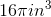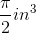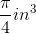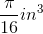Explanation:

The axle for a toy car has a length of 4 inches and a diameter of a quarter inch. What is the volume of the axle? Assume it is a cylinder.

Use the following formula for volume of a cylinder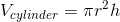Where r and h are our radius and height, respectively.

In this case, we first need to change our diameter to radius. Because our diameter is one quarter of an inch, our radius will be one eighth of an inch.

Plug it in to get: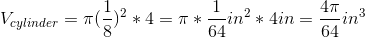Simplify to get: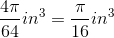### Example Question #422 : Geometry

You are visiting the drive-through at the bank. You put you money in a cylindrical tube with a height of 8 inches and a radius of 2 inches. What is the volume of the tube?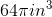Not enough information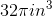Explanation:

You are visiting the drive-through at the bank. You put you money in a cylindrical tube with a height of 8 inches and a radius of 2 inches. What is the volume of the tube?

Begin with the formula for volume of a cylinder:We have r and h, which are our radius and height, respectively. Plug them in and solve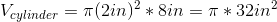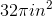### Example Question #111 : Solid Geometry

Find the volume of a cylinder with the following measurements:

• diameter = 8cm
• height = 5cm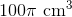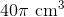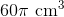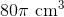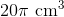Explanation:

To find the volume of a cylinder, we will use the following formula: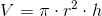where r is the radius and h is the height of the cylinder.

Now, we know the diameter of the cylinder is 8cm.  We know that the diameter is two times the radius.  Therefore, the radius is 4cm.

We also know the height of the cylinder is 5cm.

Knowing this, we can substitute into the formula.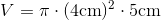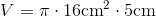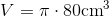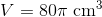### Example Question #1 : How To Find The Volume Of A Cylinder

Find the volume of a cylinder with a diameter of 8in and a height of 7in.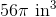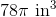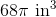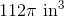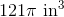Explanation:

To find the volume of a cylinder, we will use the following formula: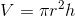where r is the radius and h is the height of the cylinder.

Now, we know the diameter of the cylinder is 8in.  We also know the diameter is two times the radius.  Therefore, the radius is 4in.

We also know the height of the cylinder is 7in.

Knowing all of this, we can substitute into the formula.  We get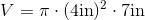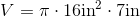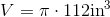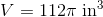### Example Question #1 : Cylinders

Find the volume of a cylinder with a diameter of 14in and a height of 12in.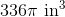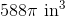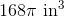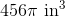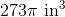Explanation:

To find the volume of a cylinder, we will use the following formula:where r is the radius, and h is the height of the cylinder.

Now, we know the diameter of the cylinder is 14in.  We also know the diameter is two times the radius.  Therefore, the radius is 7in.

We know the height of the cylinder is 12in.

Knowing all of this, we can substitute into the formula.  We get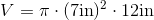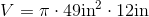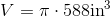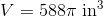### Example Question #1 : How To Find The Volume Of A Cylinder

Your family owns a farm with a silo for storing grain. If the silo is 40 feet tall and 15 feet in diameter, what volume of grain can it hold?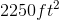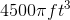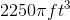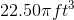Explanation:

Your family owns a farm with a silo for storing grain. If the silo is 40 feet tall and 15 feet in diameter, what volume of grain can it hold?

Begin with the formula for volume of a cylinder.A cylinder is just a circle with height.

So, we know the height is 40 ft, but what is r?

If you said 15, you would be on track to get the problem wrong. That is because the diameter is 15 ft, so our radius is only 7.5 ft.

Plug these in to get our answer: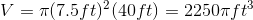### Example Question #2 : Cylinders

Let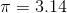Find the volume of a cylinder with a diameter of 12cm and a height of 7cm.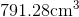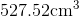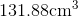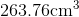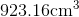Explanation:

To find the volume of a cylinder, we will use the following formula:where r is the radius and h is the height of the cylinder.

Now, we know the diameter is 12cm.  We know that the diameter is two times the radius.  Therefore, the radius is 6cm.

We know the height of the cylinder is 7cm.

We know that.

Knowing all of this, we can substitute into the formula.  We get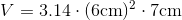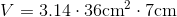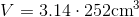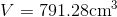### Example Question #1 : How To Find The Volume Of A Cylinder

You have a tennis ball canister which is cylindrical. If the radius of the cylinder is 3.5 cm, and the height is 21 cm, what is the volume of the canister?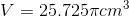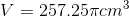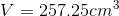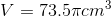Explanation:

You have a tennis ball canister which is cylindrical. If the radius of the cylinder is 3.5 cm, and the height is 21 cm, what is the volume of the canister?

Begin with the formula for volume of a cylinder:Where r is our radius and h is our height.

Note that the formula is just the formula for the area of a circle times the height, because a cylinder is really just a circle with height.

Now, plug in what we know and solve for V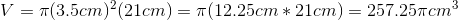### Example Question #111 : Solid Geometry

A cylinder has the following measurements:

Height: 6in
Diameter: 16in

Find the volume.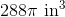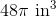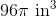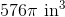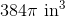Explanation:

To find the volume of a cylinder, we will use the following formula:where r is the radius and h is the height of the cylinder.

Now, we know the diameter of the cylinder is 16in.  We also know the diameter is two times the radius.  Therefore, the radius is 8in.

We also know the height of the cylinder is 6in.

Knowing all of this, we can substitute into the formula.  We get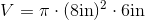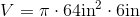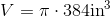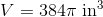### Example Question #1 : Cylinders

A cylinder has the following measurements:

Height:  12in
Diameter:  10in

Find the volume.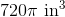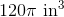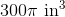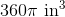Explanation:

To find the volume of a cylinder, we will use the following formula:where r is the radius, and h is the height of the cylinder.

Now, we know the diameter of the cylinder is 10in.  We also know the diameter is two times the radius.  Therefore, the radius is 5in.

We know the height of the cylinder is 12in.

Knowing all of this, we can substitute into the formula.  We get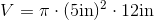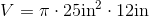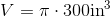← Previous 1

### All ISEE Upper Level Math Resources